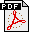Mateescu-03-a

A Generic On-the-Fly Solver for Alternation-Free Boolean Equation Systems

Proceedings of the 9th International Conference on Tools and Algorithms for the Construction and Analysis of Systems TACAS'2003 (Warsaw, Poland), April 2003

Full version available as INRIA Research Report RR-4711.

Abstract:

Boolean Equation Systems are a useful formalism for modeling various verification problems of finite-state concurrent systems, in particular the equivalence checking and the model checking problems. These problems can be solved on-the-fly (i.e., without constructing explicitly the state space of the system under analysis) by using a demand-driven construction and resolution of the corresponding boolean equation system. In this report, we present a generic software library dedicated to on-the-fly resolution of alternation-free boolean equation systems. Four resolution algorithms are currently provided by the library: A1 and A2 are general algorithms, the latter being optimized to produce small-depth diagnostics, whereas A3 and A4 are specialized algorithms for handling acyclic and disjunctive/conjunctive boolean equation systems in a memory-efficient way. The library is developed within the CADP verification toolbox and is used for both on-the-fly equivalence checking (five widely-used equivalence relations are supported) and for on-the-fly model checking of alternation-free modal mu-calculus.

 Slides of R. Mateescu presented at TACAS'03PDF Cumulated slides (in French) about CAESAR_SOLVE by R. MateescuPDF

DISCUSSION

The public presentation of this paper at TACAS'03 gave rise to several questions to which Radu Mateescu could not answer himself, since he was not present at the conference. Detailed answers to these questions follow.

Question 1 (by Moshe Vardi): It appears that Boolean Equation Systems (BES) generalize Horn clauses. It is known that for deciding HORNSAT (the satisfiability problem for Horn clauses [Shukla-Hunt-Rosenkrantz-96]), an algorithm based on a pure depth-first search (DFS) of the dependency graph such as [Dowling-Gallier-84] is not sufficient for handling cyclic dependencies [Scutella-90]. So, can a pure DFS algorithm be used for solving general BES, when it is known to fail for the HORNSAT subproblem?

Answer: It is indeed true that that BES generalize Horn clauses and that on-the-fly resolution algorithms for BESs can also be used for HORNSAT [Liu-Smolka-98]. But the Algorithm A1 of the CAESAR_SOLVE library is not simply a DFS, although it is based on a DFS of the boolean graph associated to the BES. Algorithm A1 is an optimized version of the local algorithm Avoiding 1's proposed by Andersen for the model checking of alternation-free mu-calculus [Andersen-94]. It combines a DFS with the back-propagation of stable variables (whose truth value has been decided), which allows to handle cyclic dependencies. For a detailed description of the Algorithm A1, see [Mateescu-Sighireanu-03].

References:
 [Andersen-94] H. R. Andersen. Model Checking and Boolean Graphs, Theoretical Computer Science 126(1):3-30, 1994. [Dowling-Gallier-84] W.F. Dowling and J. H. Gallier. Linear-time Algorithms for Testing the Satisfiability of Propositional Horn Formulae, Journal of Logic Programming 3:267-284. [Liu-Smolka-98] X. Liu and S. A. Smolka. Simple Linear-Time Algorithms for Minimal Fixed Points, Proceedings of ICALP'98, LNCS vol. 1443, pp. 53-66. [Scutella-90] M.G. Scutella. A note on Dowling-Gallier's Top-Down Algorithm for Propositional Horn Satisfiability, Journal of Logic Programming 8:265-273, 1990. [Shukla-Hunt-Rosenkrantz-96] S. K. Shukla, H. B. Hunt III, D. J. Rosenkrantz. HORNSAT, Model Checking, Verification and games (Extended Abstract), Proceedings of CAV'96, LNCS vol. 1102, pp. 99-110. [Mateescu-Sighireanu-03] R. Mateescu and M, Sighireanu, Efficient On-the-Fly Model-Checking for Regular Alternation-Free Mu-Calculus, Science of Computer Programming 46(3):255-281.

Question 2 (by Kim Larsen): Why don't you cite [Larsen-92], which already proposed a local BES resolution algorithm capable of producing diagnostics as modal formulas?

• State of the art efficiency is a major goal behind the design of the CAESAR_SOLVE library. All the four algorithms implemented in this library have all a (time and space) worst-case complexity linear in the size of the BES. Instead, [Andersen-94] points out that the algorithm proposed in [Larsen-92] has a quadratic worst-case complexity.

• The idea of using modal formulas for producing diagnostics was first proposed in [Cleaveland-90] and implemented in the bisimulation checker of the Concurrency Workbench. The CAESAR_SOLVE library provides a more general representation of diagnostics, which is independent from the application problem (model checking or bisimulation checking). In this approach, diagnostics are simply subgraphs of the boolean graph associated to the BES, which must be interpreted according to the application. A detailed description of the diagnostic generation features can be found in [Mateescu-00].

References:
 [Andersen-94] H. R. Andersen. Model Checking and Boolean Graphs, Theoretical Computer Science 126(1):3-30, 1994. [Cleaveland-90] R. Cleaveland. On Automatically Explaining Bisimulation Inequivalence, Proceedings of CAV'90, LNCS vol. 531, pp. 364-372. [Larsen-92] K. G. Larsen. Efficient Local Correctness Checking, Proceedings of CAV'92, LNCS vol. 663, pp. 30-43. [Mateescu-00] R. Mateescu. Efficient Diagnostic Generation for Boolean Equation Systems, Proceedings of TACAS'2000, LNCS vol. 1785, pp. 251-265.

Question 3 (by Kim Larsen): Algorithm A4 for disjunctive/conjunctive BES is claimed to have a worst-case memory complexity O (|V|), but this is only true if the right-hand sides of the equations are binary (two variables at most on the right-hand sides). Otherwise, the complexity of bisimulation checking would not depend on the number of transitions!